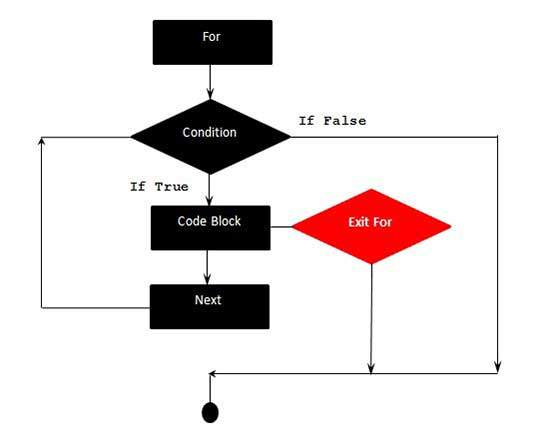# VBScript Exit For statement

A Exit For Statement is used when we want to Exit the For Loop based on certain criteria. When Exit For is executed, the control jumps to next statement immediately after the For Loop.

## Syntax

The syntax for Exit For Statement in VBScript is −

``` Exit For
```

## Flow Diagram## Example

The below example uses Exit For. If the value of the Counter reaches 4, the For Loop is Exited and control jumps to the next statement immediately after the For Loop.

```<!DOCTYPE html>
<html>
<body>
<script language = "vbscript" type = "text/vbscript">
Dim a : a = 10
For i = 0 to a Step 2 'i is the counter variable and it is incremented by 2
document.write("The value is i is : " & i)
document.write("<br></br>")

If i = 4 Then
i = i*10  'This is executed only if i = 4
document.write("The value is i is : " & i)
Exit For 'Exited when i = 4
End If
Next

</script>
</body>
</html>
```

When the above code is executed, it prints the following output in the console.

```The value is i is : 0

The value is i is : 2

The value is i is : 4

The value is i is : 40
```
vbscript_loops.htm